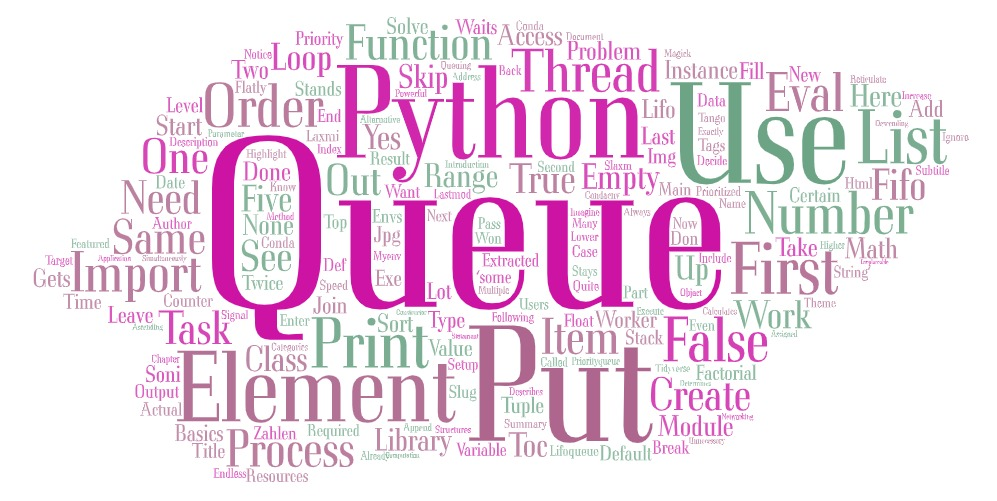# Data Science Posts and Resources

Articles on Data Science

## Python - Queues

Introduction to Python Queues

Laxmi K Soni## Queues

In Python, queues are structures that take in data in a certain order to then output it in a certain order. The default queue type is the so-called FIFO queue . This stands for first in first out and the name describes exactly what it does. The elements that enter the queue first are also the elements that will leave the queue first.

import queue

q = queue.Queue()
for x in range ( 5 ):
q.put(x)

for x in range ( 5 ):
print (q.get(x))

In order to work with queues in Python, we need to import the module queue . We can then create an instance of the class Queue by using the constructor.

As you can see, we apply two functions here – put and get . The put function adds an element to the queue that can then be extracted by the get function.

Here, we keep numbers one to five into our queue. Then, we just get the elements and print them. The order stays the same, since the default queue is FIFO .

### QUEUING RESOURCES

Let’s say we have a list of numbers that need to be processed. We decide to use multiple threads, in order to speed up the process. But there might be a problem. The threads don’t know which number has already been processed and they might do the same work twice, which would be unnecessary. Also, solving the problem with a counter variable won’t always work, because too many threads access the same variable and numbers might get skipped. In this case we can just use queues to solve our problems. We fill up our queue with the numbers and every thread just uses the get function, to get the next number and process it. Let’s assume we have the following worker function:

import threading
import queue
import math
q = queue.Queue()
def worker():
while True :
item = q.get()
if item is None :
break
print (math.factorial(item))
q.task_done()

We start out with an empty queue and an empty list for threads. Our function has an endless loop that gets numbers from the list and calculates the factorial of them. For this factorial function, we need to import the module math . But you can ignore this part, since it is only used because the computation requires a lot of resources and takes time. At the end, we use the function task_done of the queue, in order to signal that the element was processed.

for x in range ( 5 ):
t.start()

zahle = [ 1340000 , 13 , 3, 300 , 98 , 88 , 11 , 23 ]
for item in zahle:
q.put(item)
q.join()
for i in range ( 5 ):
q.put( None )

We then use a for loop to create and start five threads that we also add to our list. After that, we create a list of numbers, which we then all put into the queue.

The method join of the queue waits for all elements to be extracted and processed. Basically, it is going to wait for all the task_done functions. After that, we put None elements into the queue, so that our loops break.

Notice that our threads can’t process the same element twice or even skip one because they can only get them by using the get function. If we would use a counter for this task, two threads might increase it at the same time and then skip an element. Or they could just access the same element simultaneously. Queues are irreplaceable for tasks like this.

### LIFO QUEUES

Alternative to the FIFO queues is LIFO queues . That stands for last in first out . You can imagine this queue like some sort of stack. The element you put last on top of the stack is the first that you can get from it.

import queue
q = queue.LifoQueue()
numbers = [ 1 , 2 , 3 , 4 , 5 ]
for x in numbers:
q.put(x)
while not q.empty():
print (q.get())

By using the LifoQueue class from the queue module, we can create an instance of this type. When we now put in the numbers one to five in ascending order, we will get them back in descending order. The result would be: 5 4 3 2 1

### PRIORITIZING QUEUES

What you can also do in Python, is creating prioritized queues . In these, every element gets assigned a level of priority that determines when they will leave the queue.

import queue
q = queue.PriorityQueue()
q.put(( 8 , 'Some string' ))
q.put(( 1 , 2023 ))
q.put(( 90 , True ))
q.put(( 2 , 10.23 ))
while not q.empty():
print (q.get())

Here, we create a new instance of the class PriorityQueue . When we put a new element into this queue, we need to pass a tuple as a parameter. The first element of the tuple is the level of importance (the lower the number, the higher the priority) and the second element is the actual object or value that we want to put into the queue.

When we execute the print statement of the loop, we get the following results:

(1, 2023) (2, 10.23) (8, ‘Some string’) (90, True)

As you can see, the elements got sorted by their priority number. If you only want to access the actual value, you need to address the index one because it is the second value of the tuple. while not q.empty(): print (q.get()[ 1 ])